# Two-dimensional NMR spectroscopy

## The spin ½ nuclei seen by the quantum mechanic

### The example of the proton

The atomic physic teaches us that some nuclei have a kinetic momentum due to their spin state: P and also a magnetic momentum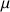, which is deﬁned by the following relation: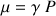where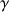is the gyro magnetic ratio, thuscharacterizes a given nucleus.

The quantum mechanic describes an atom with wave functions solution of the Schrödinger equation.

For a proton, nucleus with spin quantic number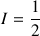, or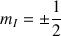the own wave functions are the following: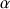for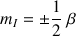for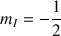.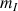corresponds to the magnetic quantic number which characterizes the stationary state of the nucleus, which depends on the spin numbers I of the nucleus. The total number of the possible own stationary states of the nucleus is then equal to : 2I+1. Thus the proton may exist from the point of view of its spin momentum in only two stationary states.

Without any magnetic ﬁeld theand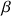have the same energy they are, so called, degenerated. It is only in a static homogenous magnetic field of value B0 and following the interaction between B0 andthat this degeneracy is suppressed (Fig.1). The separation of energy then produced is proportional to the intensity of the ﬁeld B0, and creates the necessary condition for the existence of spectroscopic transition and constitutes the basis of the spectroscopy by Nuclear Magnetic Resonance.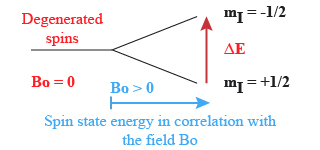Fig.1 : Energy of the spin states in corre1ation with the field Bo

The proton will occupy thestate (i.e. the weakest energy one) with a higher probability.

The more the ﬁeld B0 increases the more the difference of energy (or) between the two spin states increases. We describe the difference of occupancy between the basic state and the excitated one with the Boltzmann law :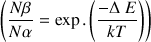Where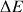is the difference of energy between the two states, k the Boltzmann constant, T the absolute temperature in °K,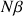the number of spins at the high energy level and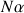the number of spins at the low energy level.

At the equilibrium only a small excess of nuclei are at the fundamental state. For a ﬁeld of 1.4 T at room temperature,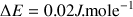. According to the theory the population excess determines the probability of excitation.

However, this population excess does not reach more than 0.001%

1. D. Canet

La RMN concepts et méthodes inter éditions 1991